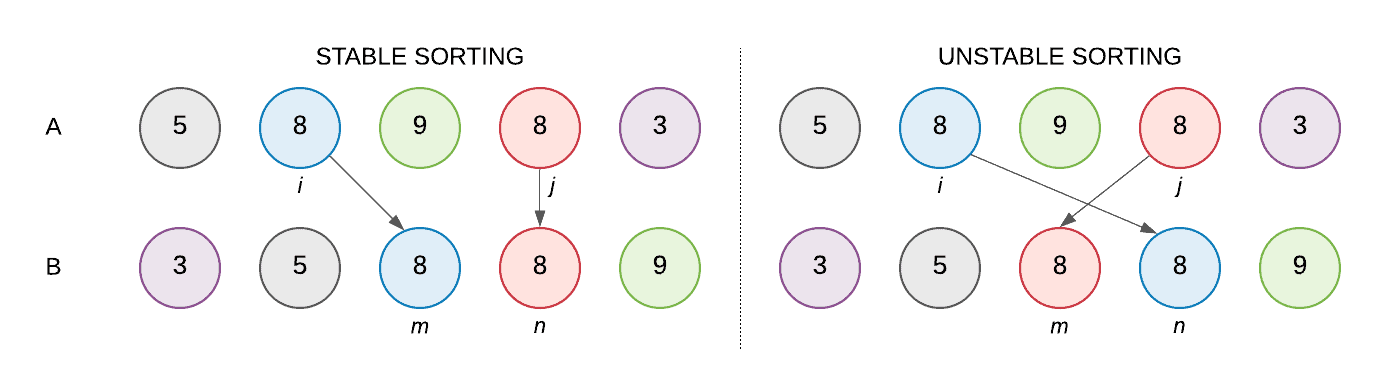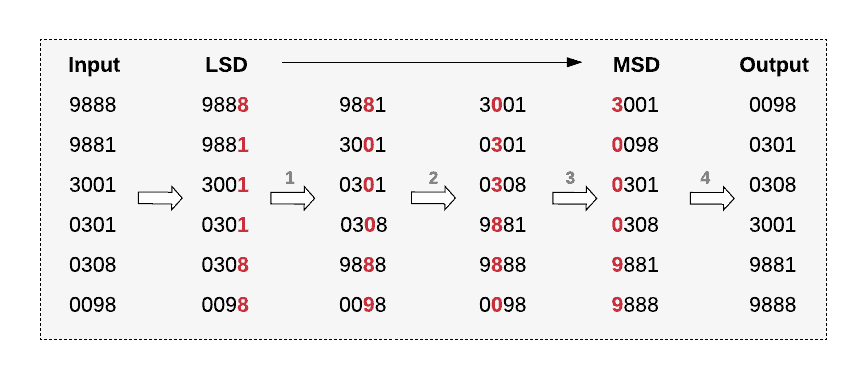### I just announced the new Learn Spring course, focused on the fundamentals of Spring 5 and Spring Boot 2:

>> CHECK OUT THE COURSE

## 1. Overview

In this tutorial, we'll learn what stable sorting algorithms are and how they work. Further, we'll explore when the stability of sorting matters.

## 2. Stability in Sorting Algorithms

The stability of a sorting algorithm is concerned with how the algorithm treats equal (or repeated) elements. Stable sorting algorithms preserve the relative order of equal elements, while unstable sorting algorithms don't. In other words, stable sorting maintains the position of two equals elements relative to one another.

Let A be a collection of elements and < be a strict weak ordering on the elements. Further, let B be the collection of elements in A in the sorted order. Let's consider two equal elements in A at indices i and j, i.e, A[i] and A[j], that end up at indices m and n respectively in B. We can classify the sorting as stable if:

```i < j and A[i] = A[j] and m < n
```

Let's understand the concept with the help of an example. We have an array of integers A:  [ 5, 8, 9, 8, 3 ]. Let's represent our array using color-coded balls, where any two balls with the same integer will have a different color which would help us keep track of equal elements (8 in our case):Stable sorting maintains the order of the two equal balls numbered 8, whereas unstable sorting may invert the relative order of the two 8s.

## 3. When Stability Matters

### 3.1. Distinguishing between Equal Elements

All sorting algorithms use a key to determine the ordering of the elements in the collection, called the sort key.

If the sort key is the (entire) element itself, equal elements are indistinguishable, such as integers or strings.

On the other hand, equal elements are distinguishable if the sort key is made up of one or more, but not all attributes of the element, such as age in an Employee class.

### 3.2. Stable Sorting is Important, Sometimes

We don't always need stable sorting. Stability is not a concern if:

• equal elements are indistinguishable, or
• all the elements in the collection are distinct

When equal elements are distinguishable, stability is imperative.  For instance, if the collection already has some order, then sorting on another key must preserve that order.

For example, let's say we are computing the word count of each distinct word in a text file. Now, we need to report the results in decreasing order of count, and further sorted alphabetically in case two words have the same count:

```Input:
how much wood would woodchuck chuck if woodchuck could chuck wood

Output:
chuck       2
wood        2
woodchuck   2
could       1
how         1
if          1
much        1
would       1
```

Once we have sorted the elements by count, we need to sort it further lexicographically. At this point, the sorting algorithm must maintain the relative order of the counts:

```First pass, sorted by count:
(wood, 2)
(chuck, 2)
(woodchuck, 2)
(much, 1)
(could, 1)
(would, 1)
(if, 1)
(how, 1)

Second pass, sorted lexicographically while preserving the previous relative order:
(chuck, 2)
(wood, 2)
(woodchuck, 2)
(could, 1)
(how, 1)
(if, 1)
(much, 1)
(would, 1)
```

Radix Sort is an integer sorting algorithm that depends on a sorting subroutine that must be stable. It is a non-comparison based sorting algorithm that sorts a collection of integers. It groups keys by individual digits that share the same significant position and value.

Let's unpack the formal definition and restate the basic idea:

```for each digit 'k' from the least significant digit (LSD) to the most significant digit (MSD) of a number:
apply counting-sort algorithm on digit 'k' to sort the input array
```

We are using Counting Sort as a subroutine in Radix Sort. Counting Sort is a stable integer sorting algorithm. We don't have to understand how it works, but that Counting Sort is stable.

Let's look at an illustrative example:Each invocation of the Counting Sort subroutine preserves the order from the previous invocations. For example, while sorting on the tens' place digit (second invocation) 9881 shifts downwards, but stays above 9888 maintaining their relative order.

Thus, Radix Sort utilizes the stability of the Counting Sort algorithm and provides linear time integer sorting.

## 4. Stable and Unstable Sorting Algorithms

Several common sorting algorithms are stable by nature, such as Merge SortTimsortCounting SortInsertion Sort, and Bubble Sort. Others such as Quicksort, Heapsort and Selection Sort are unstable.

We can modify unstable sorting algorithms to be stable. For instance, we can use extra space to maintain stability in Quicksort.

## 5. Conclusion

In this tutorial, we learned about stable sorting algorithms and looked at when stability matters, using Radix Sort as an example.

### I just announced the new Learn Spring course, focused on the fundamentals of Spring 5 and Spring Boot 2:

>> CHECK OUT THE COURSE
Notify ofGuest
Klaus

Shouldn’t lexical sort be applied first?

Though you can first sort by count, then sort all elements with the same count lexicographically, in this case specifically stability wouldn’t matter.

Sorting alphabetical first and then applying a stable sort by count across the WHOLE dataset is where stability comes in.

The first version would in theory be slightly more efficient but also make the code more complex and unviable if the sorting happens by successive global sorting in a GUI.Member
Eric Martin

Hello Klaus,
Thank you for your comment, you’re right. We’ll fix the article.
Cheers.